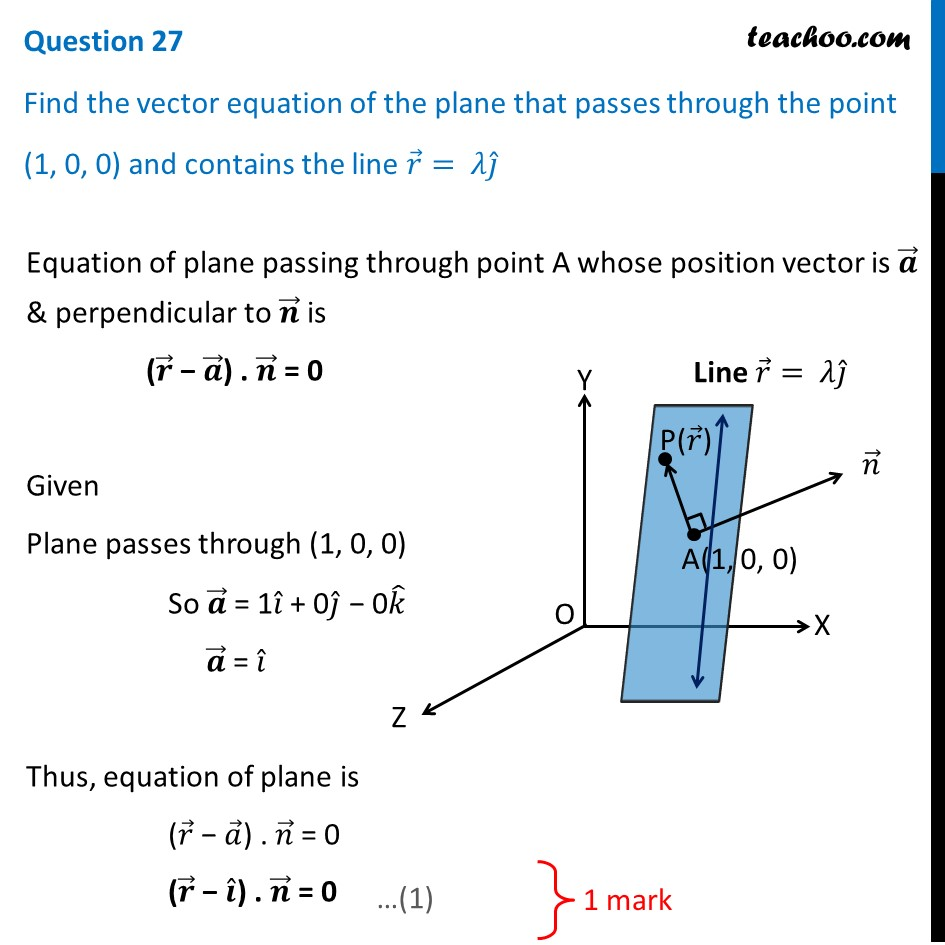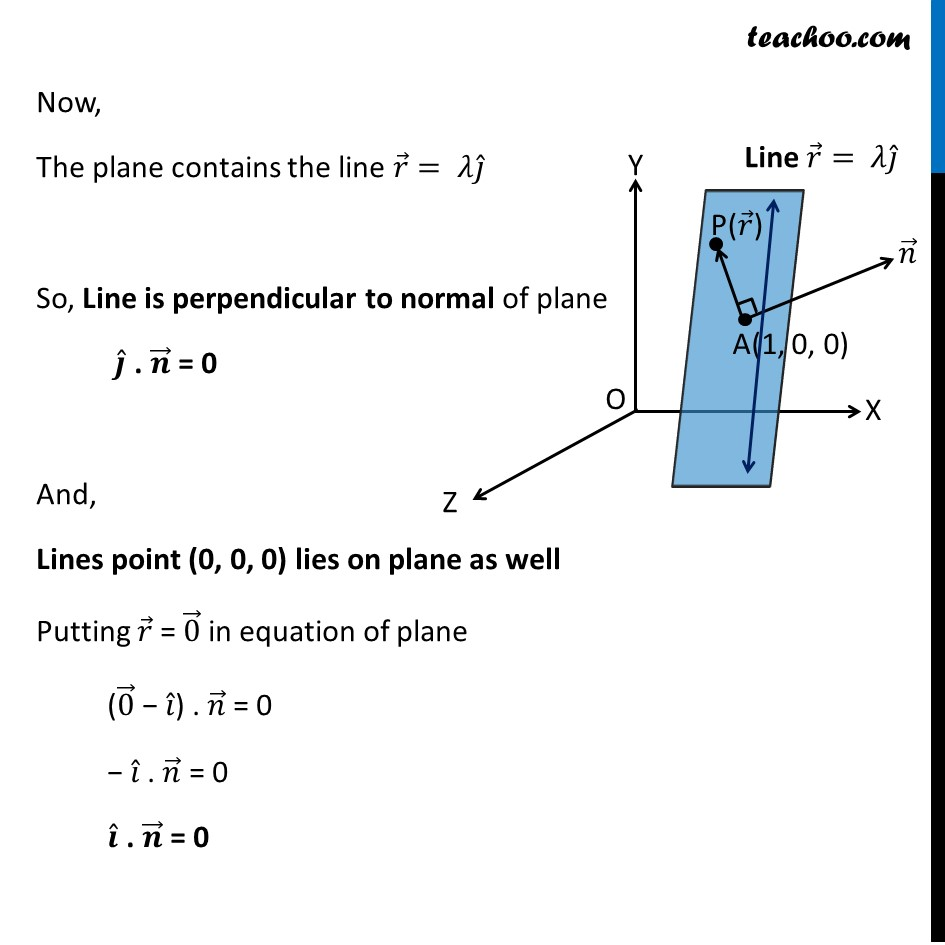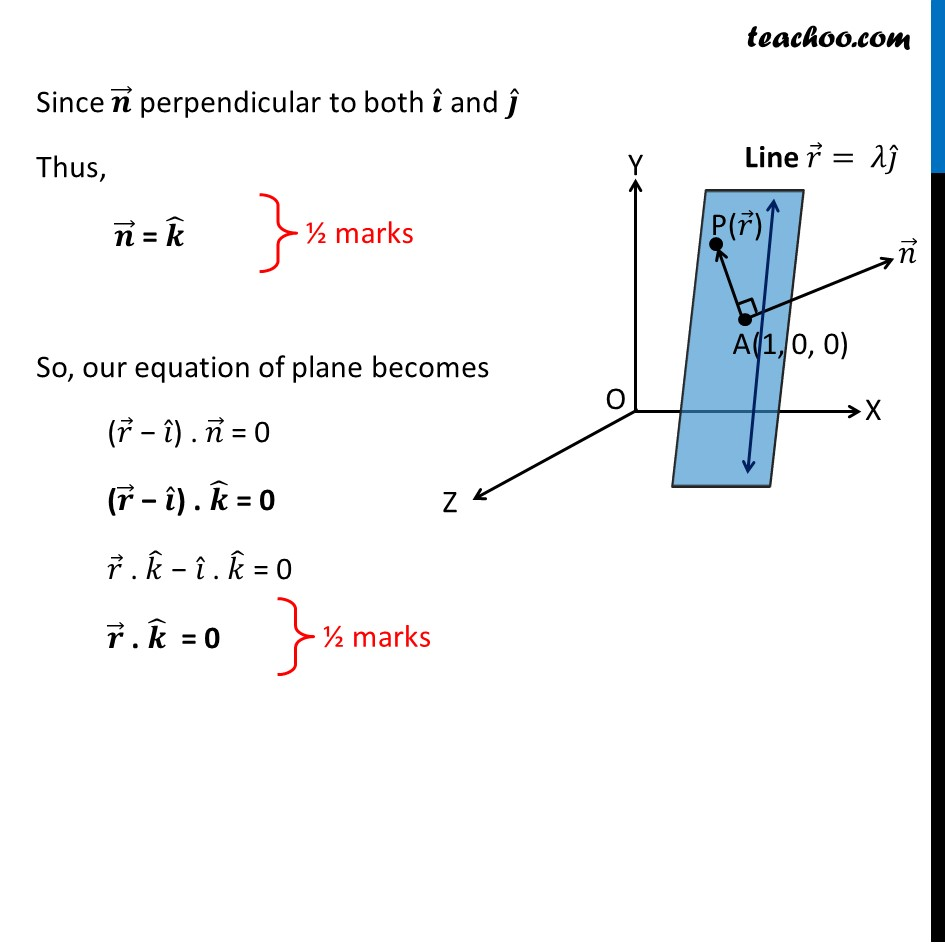## Find the vector equation of the plane that passes through the point (1, 0, 0) and contains the line r → = ⋏i ̂ j ̂1. Class 12
2. Solutions of Sample Papers and Past Year Papers - for Class 12 Boards
3. CBSE Class 12 Sample Paper for 2021 Boards

Transcript

Equation of plane passing through point A whose position vector is 𝒂 ⃗ & perpendicular to 𝒏 ⃗ is (𝒓 ⃗ − 𝒂 ⃗) . 𝒏 ⃗ = 0 Given Plane passes through (1, 0, 0) So 𝒂 ⃗ = 1𝑖 ̂ + 0𝑗 ̂ − 0𝑘 ̂ 𝒂 ⃗ = 𝑖 ̂ Thus, equation of plane is (𝑟 ⃗ − 𝑎 ⃗) . 𝑛 ⃗ = 0 (𝒓 ⃗ − 𝒊 ̂) . 𝒏 ⃗ = 0 Now, The plane contains the line 𝑟 ⃗= 𝜆𝑗 ̂ So, Line is perpendicular to normal of plane 𝒋 ̂ . 𝒏 ⃗ = 0 And, Lines point (0, 0, 0) lies on plane as well Putting 𝑟 ⃗ = 0 ⃗ in equation of plane (0 ⃗ − 𝑖 ̂) . 𝑛 ⃗ = 0 − 𝑖 ̂ . 𝑛 ⃗ = 0 𝒊 ̂ . 𝒏 ⃗ = 0 Since 𝒏 ⃗ perpendicular to both 𝒊 ̂ and 𝒋 ̂ Thus, 𝒏 ⃗ = 𝒌 ̂ So, our equation of plane becomes (𝑟 ⃗ − 𝑖 ̂) . 𝑛 ⃗ = 0 (𝒓 ⃗ − 𝒊 ̂) . 𝒌 ̂ = 0 𝑟 ⃗ . 𝑘 ̂ − 𝑖 ̂ . 𝑘 ̂ = 0 𝒓 ⃗ . 𝒌 ̂ = 0

CBSE Class 12 Sample Paper for 2021 Boards

Class 12
Solutions of Sample Papers and Past Year Papers - for Class 12 Boards